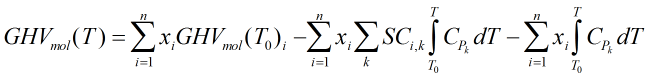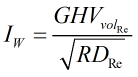# C127 – AGA 5 Natural Gas Energy Measurement

## Description

This calculates the gross and net heating values of a natural gas for custody transfer.

AGA Report No.5 – Natural Gas Energy Measurement (2009)

Kelton calculation reference C127

FLOCALC calculation reference F087

KIMS calculation reference K129

## Options

### Heating Value Method

• Molar
• Volumetric

This option allows the user to select whether they want the known values heating values of the constituent compounds to be molar or volumetric values.

### Standard Temperature

• 0°C
• 15°C
• 20°C
• 25°C
• 59°F
• 60°F

If “Molar” is selected as the Heating Value Method, this option will allow the user to select the standard temperature of the gas mixture.

### Standard Conditions

• 0°C, 100kPa
• 15°C, 101.325kPa
• 20°C, 98.0665kPa
• 59°F, 14.696psi
• 60°F, 14.4psi
• 60°F, 14.65psi
• 60°F, 14.696psi
• 60°F, 14.73psi
• 60°F, 15.025psi

If “Volumetric” is selected as the Heating Value Method, this option will allow the user to select a set of standard conditions for the gas mixture.

## Constants

Gas Constant – R = 8.314472 J/molK

Molar masses of supported constituents and air

 Gas Molar Mass (g/mol) Methane 16.0425 Ethane 30.069 Propane 44.0956 i-Butane 58.1222 n-Butane 58.1222 i-Pentane 72.1488 n-Pentane 72.1488 n-Hexane 86.1754 n-Heptane 100.2019 n-Octane 114.2285 n-Nonane 128.2551 n-Decane 142.2817 Nitrogen 28.0134 Oxygen 31.9988 Carbon Dioxide 44.0095 Carbon Monoxide 28.0101 Hydrogen Sulphide 34.0809 Water 18.0153 Hydrogen 2.0159 Helium 4.0026 Argon 39.948 2,2-Dimethyl Propane 72.1488 2-Methyl Pentane 86.1764 3-Methyl Pentane 86.1764 2,2-Dimethyl Butane 86.1764 2,3-Dimethyl Butane 86.1764 Ethylene 28.0532 Propylene 42.0797 Methyl Alcohol 32.0119 Air 28.9625

## Calculation

### Compressibility factor

The compressibility factor is calculated by:Where n = Number of constituents of natural gas mixture xi = Mole fraction of ith component of natural gas mixture si = Summation factor of ith component of natural gas mixture

### Ideal gross heating value (volumetric)

The volumetric ideal gross heating value is calculated by:Where n = Number of constituents of natural gas mixture xi = Mole fraction of ith component GHVi = Ideal gross heating value of ith component

### Gross heating value (molar)

The molar gross heating value is calculated by:Where n = Number of constituents of natural gas mixture T = Metering temperature T0 = Reference temperature xi = Mole fraction of ith component of natural gas mixture GHVmol(T)i = Molar gross heating value of ith component of natural gas mixture SCi,k = Stoichiometric coefficient of species k for combustion of ith component of natural gas mixture Cp = Molar ideal heat capacity

### Wobbe Index

The Wobbe index is calculated byWhere RDRe = Relative density of natural gas mixture GHVVolRe = Real gas volumetric gross heating value of natural gas mixture

Back